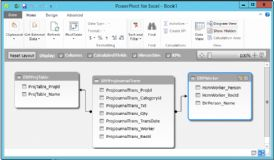# PowerPivot With Excel - Level 1

Enquire About This CourseWe are currently delivering Online & Classroom Power Pivot training - Classroom Based Training is delivered at our Belfast City Centre Premises in Amelia Street (based just opposite the Europa Hotel):

# PowerPivot with Excel Training Courses in Belfast NI

PowerPivot with Excel Level 1

PREREQUISITES

• Demonstrate a good working knowledge of Microsoft Excel
• Understand formula/function writing to manipulate data
• Understand basic features of Pivot Tables, such as the area designed for fields

SUMMARY

This course takes Pivot Tables to the next level.  You will learn how to use PowerPivot to access data sources, create relationships, PowerPivot DAX Expressions and Format reports; enabling deeper business insight and shorter decision making cycles.

LEARNING OUTCOMES

• Understand the basic principles of data modelling
• Better understand how PowerPivot might be applied back in your workplace
• Use some of the most common DAX functions to extend the power of the Power Pivot data model
• You will be able to communicate business and data insights with dashboards

COURSE CONTENT

Introduction

• What is PowerPivot?
• What is a PivotTable?
• Example with classical Excel PivotTable
• First Steps with PowerPivot
• Example with PowerPivot

Basic PowerPivot Concepts

• Handling Technical and Useless Columns
• Understanding Calculated Columns
• Formatting Numbers
• Using Lookup Tables
• Understanding Calculated Fields
• Handling Many Tables
• Refreshing Data
• Using SlicersPower View

• Creating a Power View Report
• Inserting New Views in Power View
• Type of Charts
• Applying Filters to Power View ReportsUnderstanding Data Models

• What is a Data Model?
• Why do PowerPivot Users need Data Modelling?
• Normalization and Denormalization
• Empty and Default Values

Introduction to DAX

• Calculation Foundations Calculated Columns Examples
• DAX Data Types
• DAX Operators
• Calculated Fields Examples
• Choosing Between Calculated Columns and Calculated Fields
• Common DAX Functions Examples Why a Calendar Table is Useful
• Logical Functions
• Mathematical Functions
• Text Functions
• Date and Time Functions

Time Intelligence in PowerPivot

• Why a Calendar Table is Useful
• Creating a Calendar Table with Excel
• Excel Tips to Create a Calendar